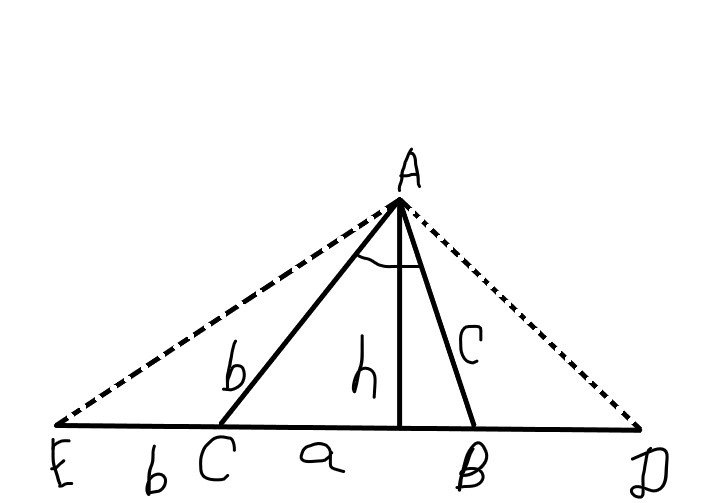# Let the angle at vertex A be called alpha. Polya says by looking at the diagram and the isoceles triangles triangle ACE and triangle ABD we can deduce that angle DAE=alpha/2+90^circ.deiluefniwf 2022-09-16 Answered
How is this angle measured in the triangle?
Given the image below:Let the angle at vertex A be called $\alpha$. Polya says by looking at the diagram and the isoceles triangles $\mathrm{△}ACE$ and $\mathrm{△}ABD$ we can deduce that $\mathrm{\angle }DAE=\frac{\alpha }{2}+90°$.
Where will I get $90°$ from?
You can still ask an expert for help

• Live experts 24/7
• Questions are typically answered in as fast as 30 minutes
• Personalized clear answers

Solve your problem for the price of one coffee

• Math expert for every subject
• Pay only if we can solve itdevilvunga
Step 1
$\mathrm{\angle }DAE=\mathrm{\angle }EAC+\mathrm{\angle }DAB+\alpha$
$=\frac{180°-\mathrm{\angle }ACE}{2}+\frac{180°-\mathrm{\angle }ABD}{2}+\alpha$
$=\frac{\mathrm{\angle }ACB+\mathrm{\angle }ABC}{2}+\alpha$
Step 2
$=\frac{180°-\alpha }{2}+\alpha$
$=90°+\frac{\alpha }{2}$

We have step-by-step solutions for your answer!Hana Buck
Step 1
Exterior angle of triangle = sum of 2 interior opposite angles
$\mathrm{\angle }ACB=2\mathrm{\angle }EAC\phantom{\rule{0ex}{0ex}}\mathrm{\angle }ABC=2\mathrm{\angle }DAB\phantom{\rule{0ex}{0ex}}$
Step 2
$⇒\mathrm{\angle }EAC+\mathrm{\angle }DAB=0.5\left(\mathrm{\angle }ACB+\mathrm{\angle }ABC\right)=0.5\left(180-\alpha \right)$
$\mathrm{\angle }DAE=90-0.5\alpha +\alpha$

We have step-by-step solutions for your answer!